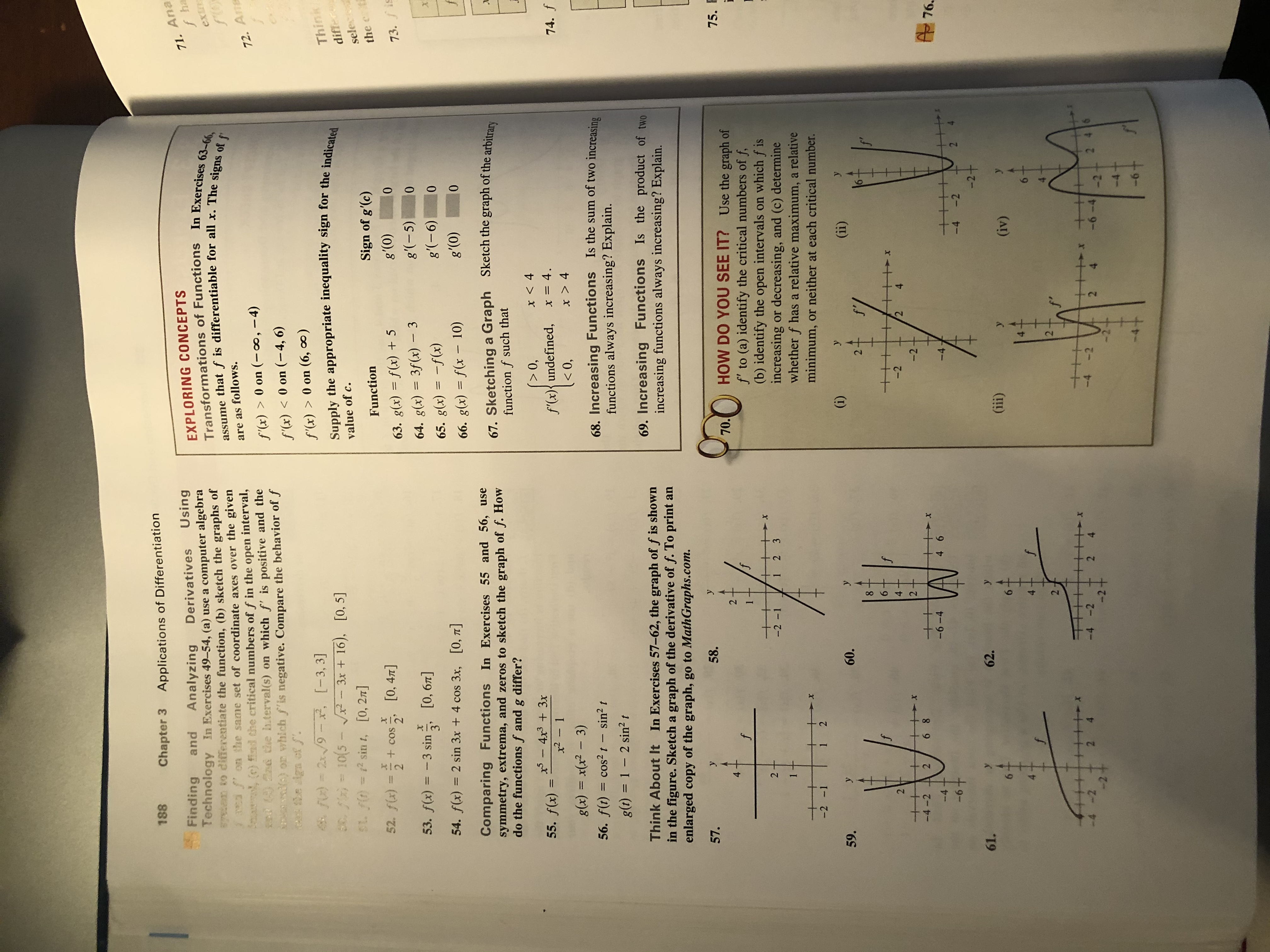# 188Chapter 3Applications of Differentiation71. Anaf haFindingTechnology In Exercises 49-54, (a) use a computer algebraan to diferentiate the function, (b) sketch the graphs of2 3PEA om the same set of coordinate axes over the givenSma (c) find the critical numbers of f in the open interval,18 Gad chhe h.terval(s) on which f' is positive and the)or which f'is negative. Compare the behavior of fca he ign offUsingandAnalyzingDerivativesEXPLORING CONCEPTSTransformations of Functions In Exercises 63-66,assume that f is differentiable for all x. The signs of fexinare as follows.72. Anf'(x) > 0 on (-0, -4)f'(x) < 0 on (-4, 6)f'(x)> 0 on (6, oo)48 FO) = 2x/9- x, [-3, 3]0 /) = 10(5 - 3x+ 16),ThinkdifferSupply the appropriate inequality sign for the indicated[0, 5]value of c.SL f(D =2 sin t, [0, 2]seleSign of g'(c)Function52. f)=2-+cos [0, 47]the+COSg'(0)1063. g(x) f(x) +573.fisg'(-5)53. f(x) 3 sin064. g(x) 3f(x) - 3[0, 67]g'(-6)065. g(x)f(x)54. f(x) 2 sin 3x + 4 cos 3x, [0, 7T]'(0)066. g(x) = f(x - 10)Comparing FunctionsIn Exercises 55 and 56, usesymmetry, extrema, and zeros to sketch the graph of f. Howdo the functions f and g differ?Sketch the graph of the arbitrary67. Sketchinga Graphfunction f such that-4x3 3x0,x < 455. f(x) =f'(x){undefined,x2- 1x = 4.74. f 4g(x) = x(x2 - 3)56. f(t) cos2t- sin2 tIs the sum of two increasing68. Increasing Functionsfunctions always increasing? Explain.11 - 2 sin2 tg(t)69. Increasing Functions Is the product of twoincreasing functions always increasing? Explain.Think About It In Exercises 57-62, the graph of f is shownin the figure. Sketch a graph of the derivative of f. To print anenlarged copy of the graph, go to MathGraphs.com57.y58.y. HOW DO YOU SEE IT? Use the graph off' to (a) identify the critical numbers of f(b) identify the open intervals on which f isincreasing or decreasing, and (c) determinewhether f has a relative maximum, a relativeminimum, or neither at each critical number.75.42ff1++2 --2 -112 31++-2 -1+1 2(i)59.y60.(ii)f'2.f624-224+-4-22-2-6 8A 76.-6-4-4+4 6-4-6+2-4-2-2+61.62.y(ii)6-(iv)422 4-4 -22 4-22 462 4-2 +-6-4-2-6+4t22

Question
1 views

58help_outlineImage Transcriptionclose188 Chapter 3 Applications of Differentiation 71. Ana f ha Finding Technology In Exercises 49-54, (a) use a computer algebra an to diferentiate the function, (b) sketch the graphs of 2 3PEA om the same set of coordinate axes over the given Sma (c) find the critical numbers of f in the open interval, 18 Gad chhe h.terval(s) on which f' is positive and the )or which f'is negative. Compare the behavior of f ca he ign off Using and Analyzing Derivatives EXPLORING CONCEPTS Transformations of Functions In Exercises 63-66, assume that f is differentiable for all x. The signs of f exin are as follows. 72. An f'(x) > 0 on (-0, -4) f'(x) < 0 on (-4, 6) f'(x)> 0 on (6, oo) 48 FO) = 2x/9- x, [-3, 3] 0 /) = 10(5 - 3x+ 16), Think differ Supply the appropriate inequality sign for the indicated [0, 5] value of c. SL f(D =2 sin t, [0, 2] sele Sign of g'(c) Function 52. f)=2 -+cos [0, 47] the +COS g'(0) 10 63. g(x) f(x) +5 73.fis g'(-5) 53. f(x) 3 sin 0 64. g(x) 3f(x) - 3 [0, 67] g'(-6) 0 65. g(x)f(x) 54. f(x) 2 sin 3x + 4 cos 3x, [0, 7T] '(0) 0 66. g(x) = f(x - 10) Comparing FunctionsIn Exercises 55 and 56, use symmetry, extrema, and zeros to sketch the graph of f. How do the functions f and g differ? Sketch the graph of the arbitrary 67. Sketchinga Graph function f such that -4x3 3x 0, x < 4 55. f(x) = f'(x){undefined, x2- 1 x = 4. 74. f <0, x > 4 g(x) = x(x2 - 3) 56. f(t) cos2t- sin2 t Is the sum of two increasing 68. Increasing Functions functions always increasing? Explain. 1 1 - 2 sin2 t g(t) 69. Increasing Functions Is the product of two increasing functions always increasing? Explain. Think About It In Exercises 57-62, the graph of f is shown in the figure. Sketch a graph of the derivative of f. To print an enlarged copy of the graph, go to MathGraphs.com 57. y 58. y . HOW DO YOU SEE IT? Use the graph of f' to (a) identify the critical numbers of f (b) identify the open intervals on which f is increasing or decreasing, and (c) determine whether f has a relative maximum, a relative minimum, or neither at each critical number. 75. 4 2 f f 1 ++ 2 - -2 -1 1 2 3 1 ++ -2 -1 + 1 2 (i) 59. y 60. (ii) f' 2. f 6 2 4 -2 2 4 + -4-2 2 -2- 6 8 A 76. -6-4 -4+ 4 6 -4 -6+ 2 -4 -2 -2+ 61. 62. y (ii) 6- (iv) 4 2 2 4 -4 -2 2 4 -2 2 46 2 4 -2 + -6-4 -2 -6+ 4t 2 2 fullscreen
check_circle

Step 1

To sketch the graph of the derivative of f(x) , given the graph of f(x)

Step 2

The graph of y=f(x) is a straight line , with (constant) slope 2. so the derivat...

### Want to see the full answer?

See Solution

#### Want to see this answer and more?

Solutions are written by subject experts who are available 24/7. Questions are typically answered within 1 hour.*

See Solution
*Response times may vary by subject and question.
Tagged in

### Derivative